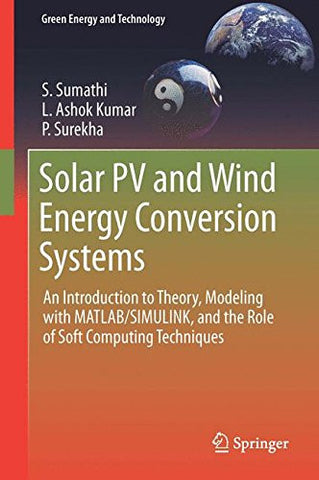Shopping Cart### Solar PV and Wind Energy Conversion Systems: An Introduction to Theory, Modeling with MATLAB/SIMULINK, and the Role of Soft Computing Techniques (Green Energy and Technology)

Regular price \$160.02 Sale price

Compare at \$194.19

• ISBN-13: 9783319149400
• Publisher: Springer
• Release Date: Apr 09, 2015
• Edition: 2015
• Pages: 790 pages
• Dimensions: 1.69 x 9.21 x 6.14 inches

### Overview

This textbook starts with a review of the principles of operation, modeling and control of common solar energy and wind-power generation systems before moving on to discuss grid compatibility, power quality issues and hybrid models of Solar PV and Wind Energy Conversion Systems (WECS). MATLAB/SIMULINK models of fuel cell technology and associated converters are discussed in detail. The impact of soft computing techniques such as neural networks, fuzzy logic and genetic algorithms in the context of solar and wind energy is explained with practical implementation using MATLAB/SIMULINK models.

This book is intended for final year undergraduate, post-graduate and research students interested in understanding the modeling and control of Solar PV and Wind Energy Conversion Systems based on MATLAB/SIMULINK.

- Each chapter includes “Learning Objectives” at the start, a “Summary” at the end and helpful Review Questions

- Includes MATLAB/SIMULINK models of different control strategies for power conditioning units in the context of Solar PV

- Presents soft computing techniques for Solar PV and WECS, as well as MATLAB/SIMULINK models, e.g. for wind turbine topologies and grid integration

- Covers hybrid solar PV and Wind Energy Conversion Systems with converters and MATLAB/SIMULINK models

- Reviews harmonic reduction in Solar PV and Wind Energy Conversion Systems in connection with power quality issues

- Covers fuel cells and converters with implementation using MATLAB/SIMULINK